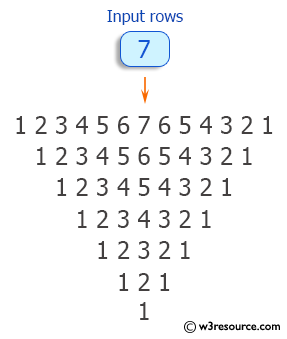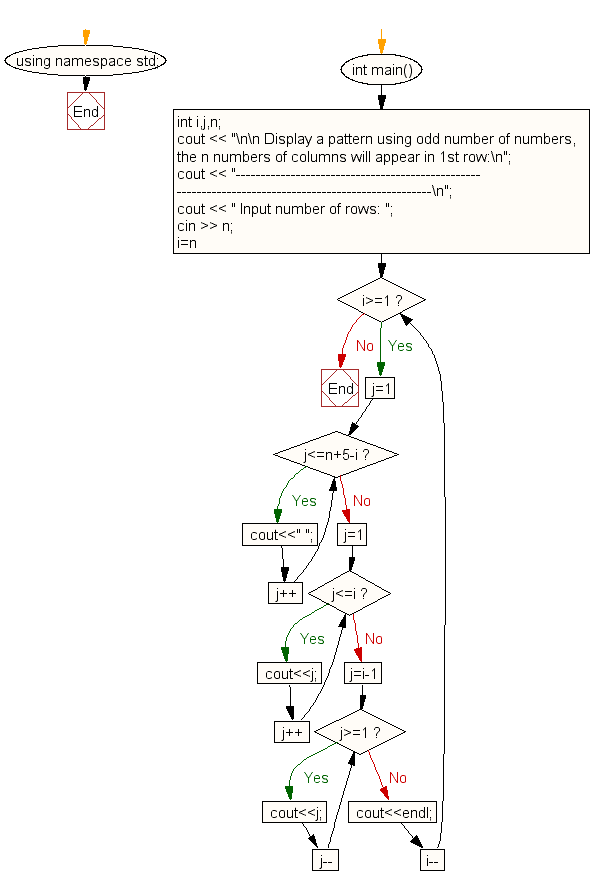﻿ C++ : Display pattern for n number of rows using number# C++ Exercises: Display specified pattern for n number of rows using number

## C++ For Loop: Exercise-55 with Solution

Write a program in C++ to display such a pattern for n number of rows using number. Each row will contain odd numbers of number. The first and last number of each row will be 1 and middle column will be the row number. n numbers of columns will appear in 1st row.

``` Input number of rows: 7
1234567654321
12345654321
123454321
1234321
12321
121
1 ```

Pictorial Presentation:Sample Solution:-

C++ Code :

``````#include <iostream>
using namespace std;

int main()
{
int i,j,n;
cout << "\n\n Display a pattern using odd number of numbers, the n numbers of columns will appear in 1st row:\n";
cout << "----------------------------------------------------------------------------------------------------\n";
cout << " Input number of rows: ";
cin >> n;
for(i=n;i>=1;i--)
{
/* print blank spaces */
for(j=1;j<=n+5-i;j++)
cout<<" ";
/* Display number in ascending order upto middle*/
for(j=1;j<=i;j++)
cout<<j;

/* Display  number in reverse order after middle */
for(j=i-1;j>=1;j--)
cout<<j;
cout<<endl;
}
}
``````

Sample Output:

``` Display a pattern using odd number of numbers, the n numbers of column
s will appear in 1st row:
-----------------------------------------------------------------------
-----------------------------
Input number of rows: 7
1234567654321
12345654321
123454321
1234321
12321
121
1
```

Flowchart:C++ Code Editor:

Contribute your code and comments through Disqus.

What is the difficulty level of this exercise?

﻿